This post describes my paper Scalable methods for computing state similarity in deterministic MDPs, published at AAAI 2020. The code is available here.

## Motivation

We consider distance metrics between states in an MDP. Take the following MDP, where the goal is to reach the green cells: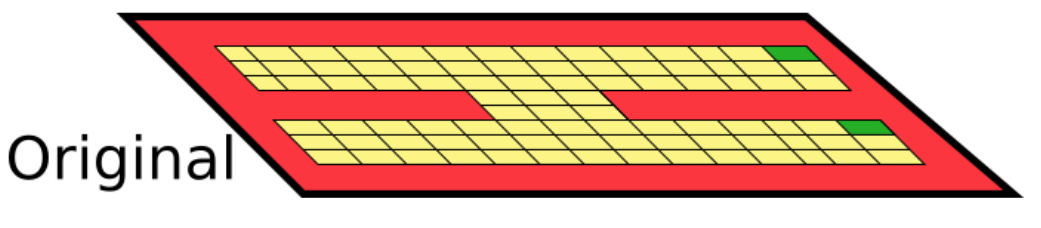### Physical distance betweent states?

Physical distance often fails to capture the similarity properties we’d like: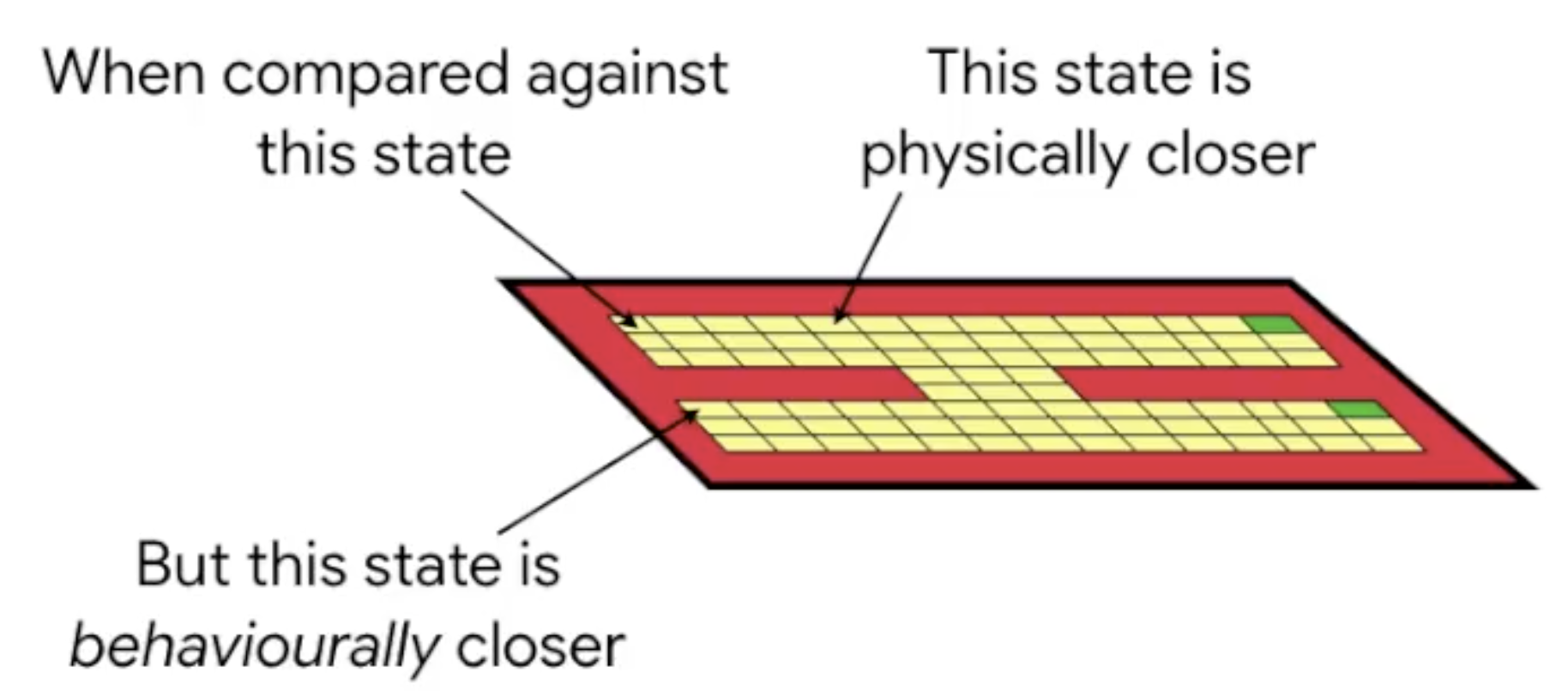### State abstractions

Now imagine we add an exact copy of these states to the MDP (think of it as an additional “floor”):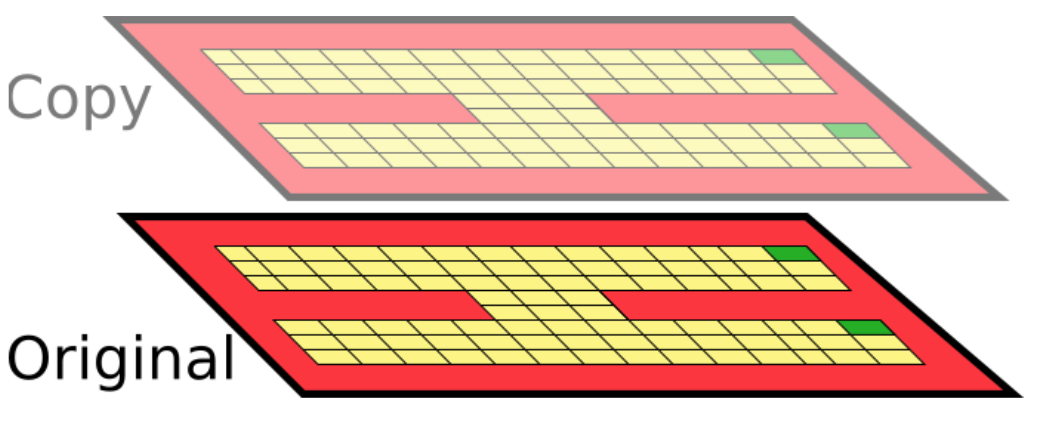And imagine after each action the agent randomly transitions between these two floors: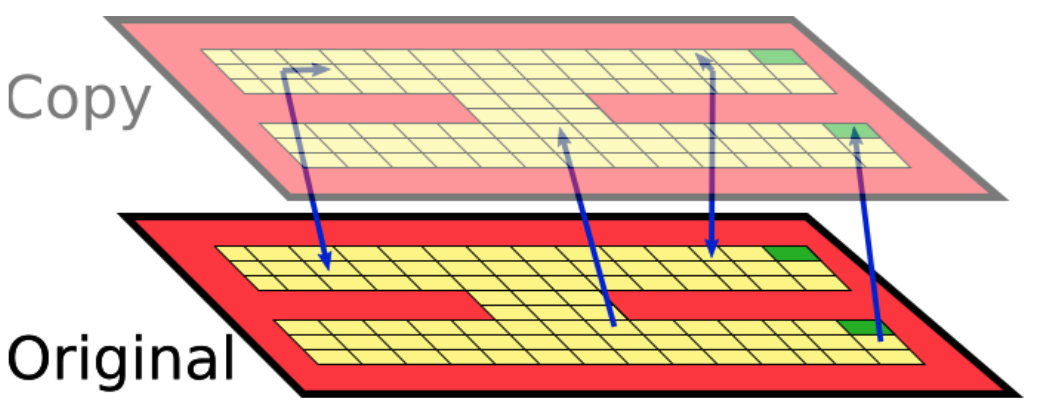Notice that since the optimal policy is identical on both floors, we just doubled the state space for no reason! We should really be grouping together similar states.

## MDPs

Consider the standard MDP defintion $\langle\mathcal{S}, \mathcal{A}, \mathcal{R}, \mathcal{P}, \gamma\rangle$, where $\mathcal{S}$ is the state space, $\mathcal{A}$ is the action space, $\mathcal{R}:\mathcal{S}\times\mathcal{A}\rightarrow\mathbb{R}$ is the one-step reward function, $\mathcal{P}:\mathcal{S}\times\mathcal{A}\rightarrow\Delta(\mathcal{S})$ is the next-state distribution function, and $\gamma\in [0, 1)$ is the discount factor.

## Bisimulation

What we need is a notion of state distance that captures behavioural indistinguishability.

### Equivalence relations

First, let me explain bisimulation relations, which was introduced by Givan, Dean, and Greig.

Two states, $s$ and $t$, are considered bisimlar (denoted $s\sim t$) if, under all actions:

1. They have equal immediate rewards: $$\forall a\in\mathcal{A}.\quad \mathcal{R}(s, a) = \mathcal{R}(t, a)$$
2. Transition with equal probability to bisimulation equivalence classes: $$\forall a\in\mathcal{A}, \forall c\in\mathcal{S}/_{\sim}.\quad\mathcal{P}(s, a)(c) := \sum_{s’\in c}\mathcal{P}(s, a)(s’) = \mathcal{P}(t, a)(c)$$

This is often easiest to understand via an illustration. Consider the folloowing system: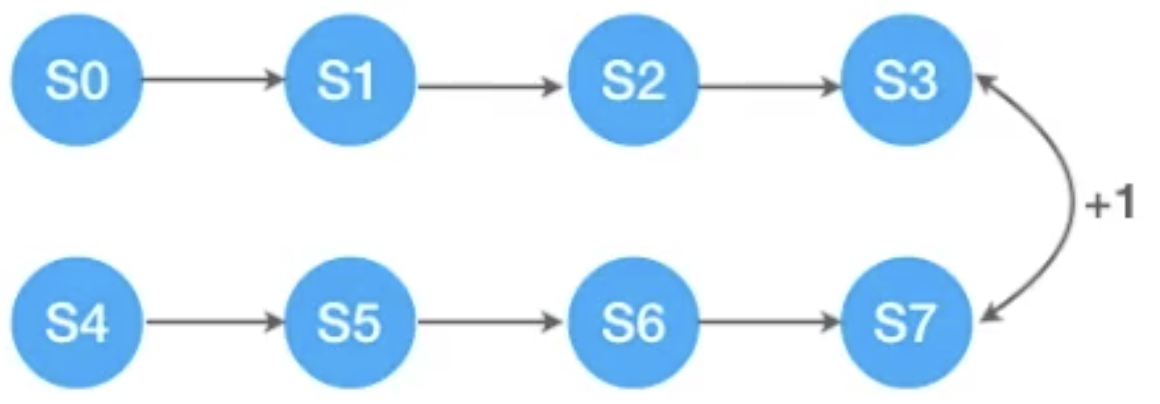A bisimulation equivalence relation would collapse the 8 states above into an equivalent 4-state MDP: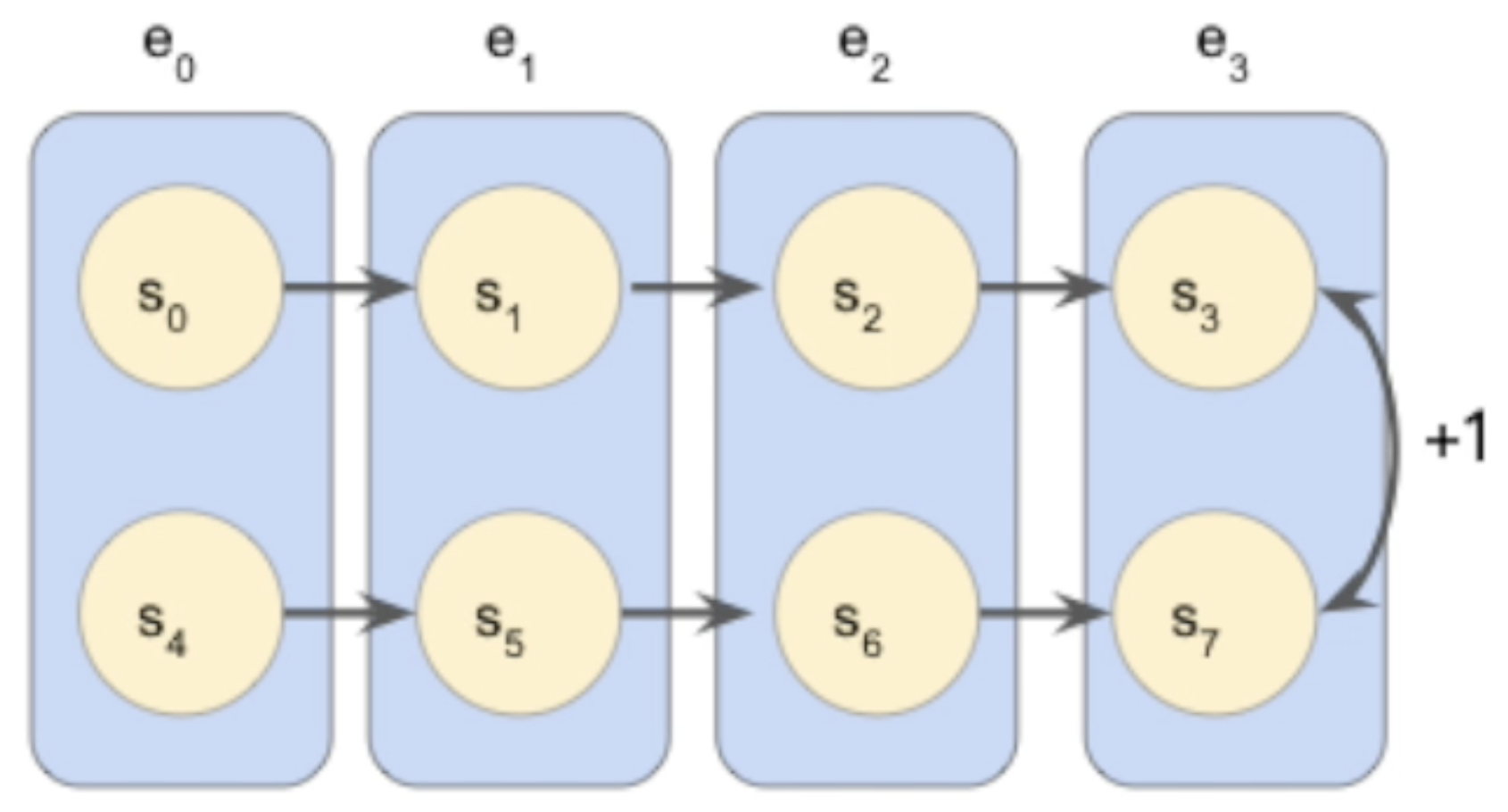But, unfortunately, bisimulation equivalence is brittle, as it’s a 0/1 relationship!

### Metrics

Bisimulation metrics generalize bisimulation relations, and quantify the behavioural distance between two states in an MDP. They are defined as any metric $d$ where $d(s, t) = 0 \iff s\sim t$. Ferns, Panangaden, and Precup proved that the following operator admits a fixed point $d^{\sim}$, and this fixed point is a bisimulation metric:

$$F(d)(s, t) = \max_{a\in\mathcal{A}}\left[ |R(s, a) - R(t, a)| + \gamma T_K(d)(P(s, a), P(t, a)) \right]$$

where $s,t\in\mathcal{S}$ are two states in the MDP, $\mathcal{A}$ is the action space, $R:\mathcal{S}\times\mathcal{A}\rightarrow\mathbb{R}$ is the reward function, $P:\mathcal{S}\times\mathcal{A}\rightarrow\Delta(\mathcal{S})$ is the transition function, and $T_K(d)$ is the Kantorovich (also known as the Wasserstein, Earth Mover’s, Sinkhorn, …) distance between two probability distributions under a state metric $d$.

These metrics have nice theoretical properties, such as: the bisimulation distance between two states is an upper-bound on their optimal value difference:

$$| V^*(s) - V^*(t)| \leq d^{\sim}(s, t)$$

## Shortcomings and solutions

Bisimulation metrics have three shortcomings I address in my paper.

### Pessimism

#### Problem

They’re inherently pessimistic (the max is considering the worst possible case!): $$F(d)(s, t) = {\color{red} \max_{a\in\mathcal{A}}}\left[ |R(s, a) - R(t, a)| + \gamma T_K(d)(P(s, a), P(t, a)) \right]$$ Take the following example MDP, where edge labels indicate the action (${a, b}$) and non-zero rewards ($[K]$).When $\gamma = 0.9$, $V^∗(s) = V^∗(t) = 10K$, while $d^{\sim}(s, t) = 10K$, which shows that the bound mentioned in the last section can be made to be as loose as desired.

#### Solution

I introduce on-policy bisimulation metrics, $d^{\pi}_{\sim}$. These are similar to regular bisimulation metrics, but are defined with respect to a policy $\pi:\mathcal{S}\rightarrow\Delta(\mathcal{A})$. In the paper I prove that $$|V^{\pi}(s) - V^{\pi}(t) | \leq d^{\pi}_{\sim}(s, t)$$

### Computational Expense

#### Problem

Bisimulation metrics have been traditionally solved via dynamic programming. But this can be very expensive, as it requires updating the metric estimate for all state-action pairs at every iteration! Note that this means computing the Kantorovich (which is an expensive linear program) $|\mathcal{S}|\times|\mathcal{A}|$ times at each iteration.

#### Solution

Sampling! If we assume a deterministic MDP, I provide an update rule using sampled pairs of $\langle s, a, r, s’\rangle$ transitions, and prove that this is guaranteed to converge to the true metric! See Theorem 4 in the paper for details.

### Full state enumerability

#### Problem

Existing methods for computing/approximating bisimulation metrics require full state enumerability.

#### Solution

Use neural networks! Consider a trained Rainbow agent, and take the penultimate layer as the representation ($\phi$):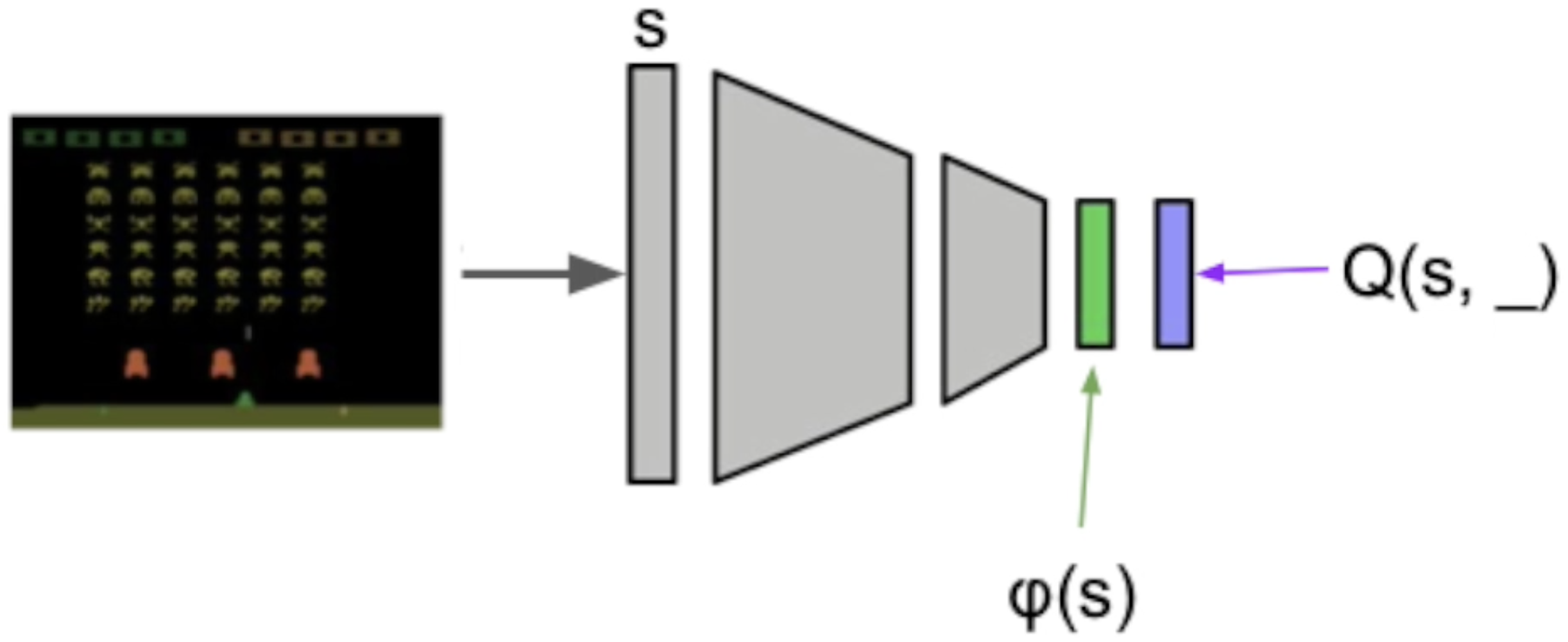We can concatenate the representations of two states: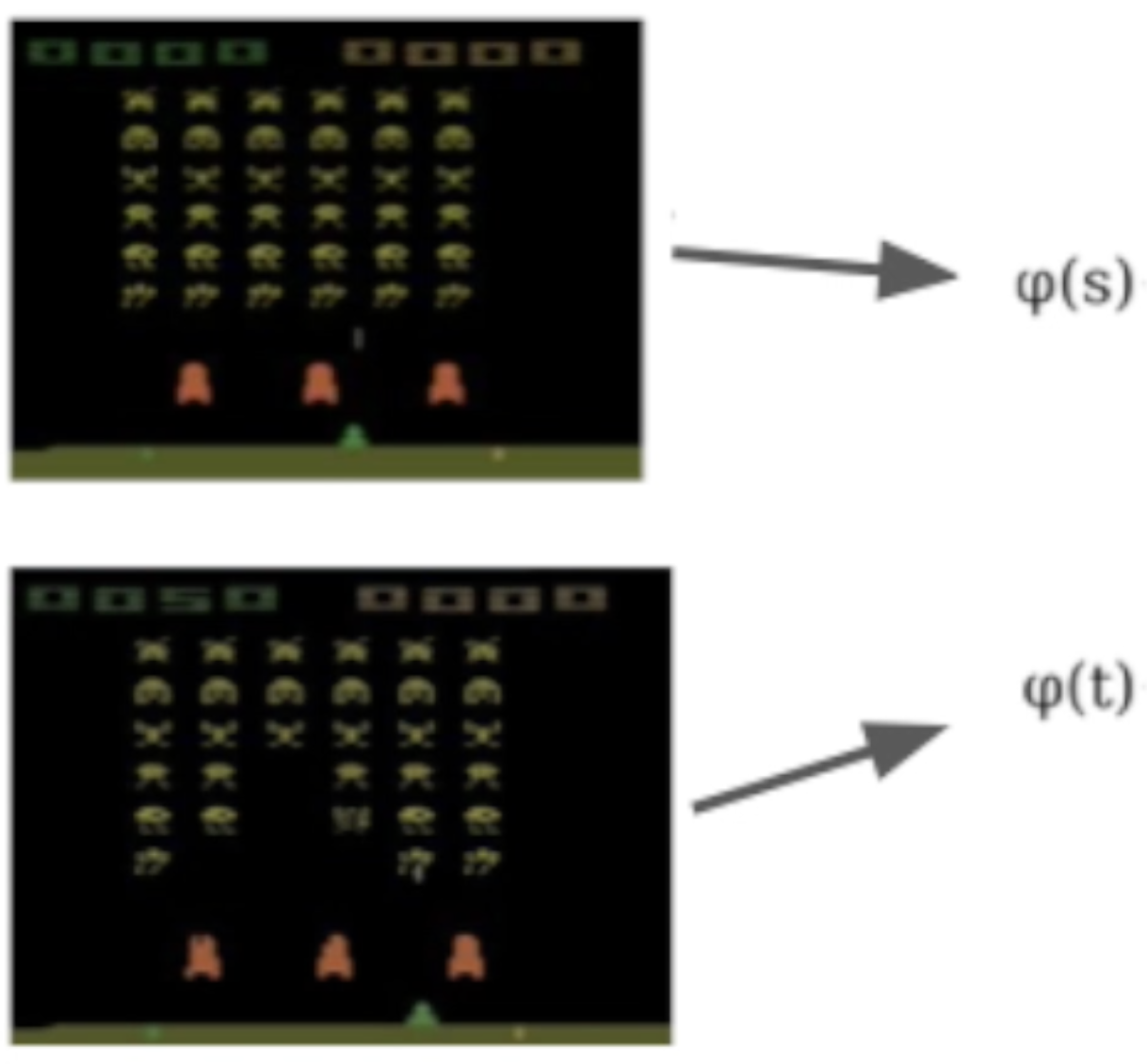And feed them through a neural network, where we denote the output of the network as $\psi(s, t)$: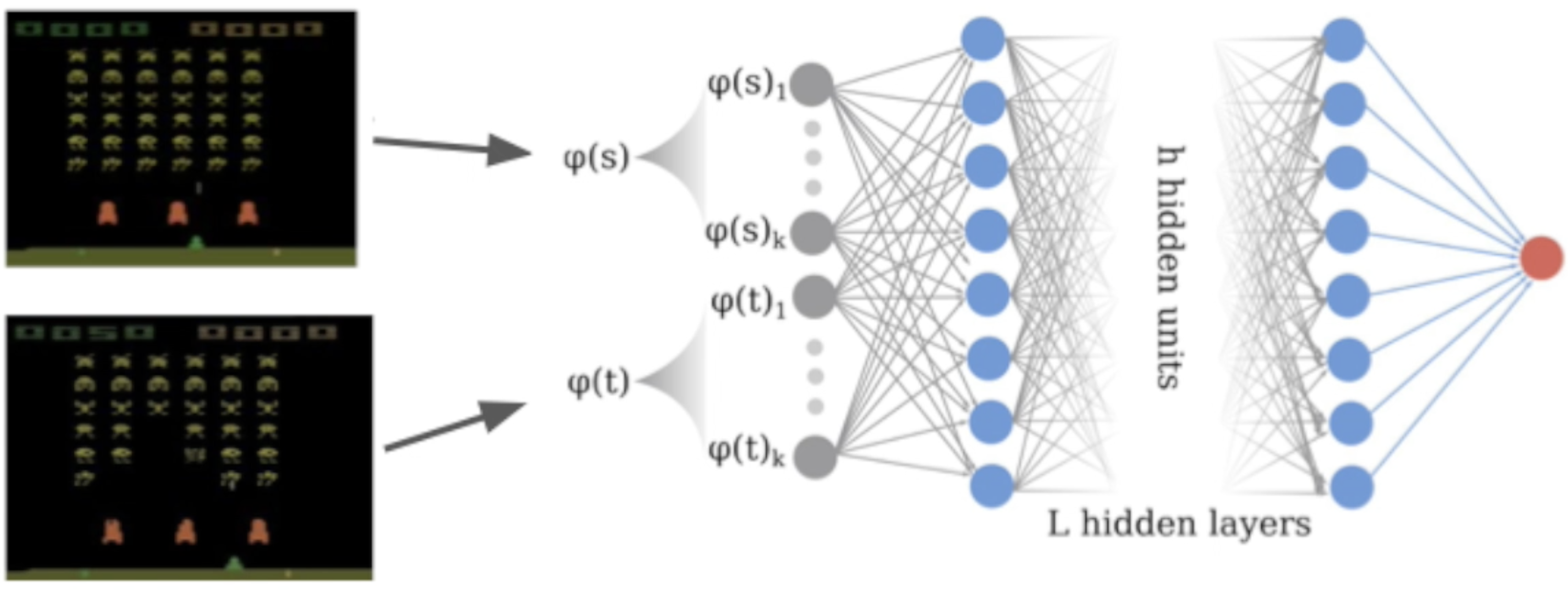We define a target for regular bisimulation ($s’$ and $t’$ are the unique next states from $s$ and $t$, respectively): $$\mathbf{T}_{\theta}(s, t, a) = \max (\psi_{\theta}(s, t, a), |\mathcal{R}(s, a)-\mathcal{R}(t, a)| + \gamma\psi_{\theta}(s’, t’))$$

and for on-policy bisimulation: $$\mathbf{T}^{\pi}_{\theta}(s, t, a) = |\mathcal{R}^{\pi}(s)-\mathcal{R}^{\pi}(t)| + \gamma\psi^{\pi}_{\theta}(s’, t’))$$

Which is then incorporated into the loss (where $\mathcal{D}$ is the dataset, e.g. a replay buffer): $$\mathcal{L}^{(\pi)}_{s,t,a} = \mathbb{E}_{\mathcal{D}}\left(\mathbf{T}^{(\pi)}_{\theta}(s, t, a) - \psi^{(\pi)}_{\theta}(s, t, a)\right)^2$$

## Evaluate on Atari 2600

Take a trained Rainbow agent and compute the distance from the first state: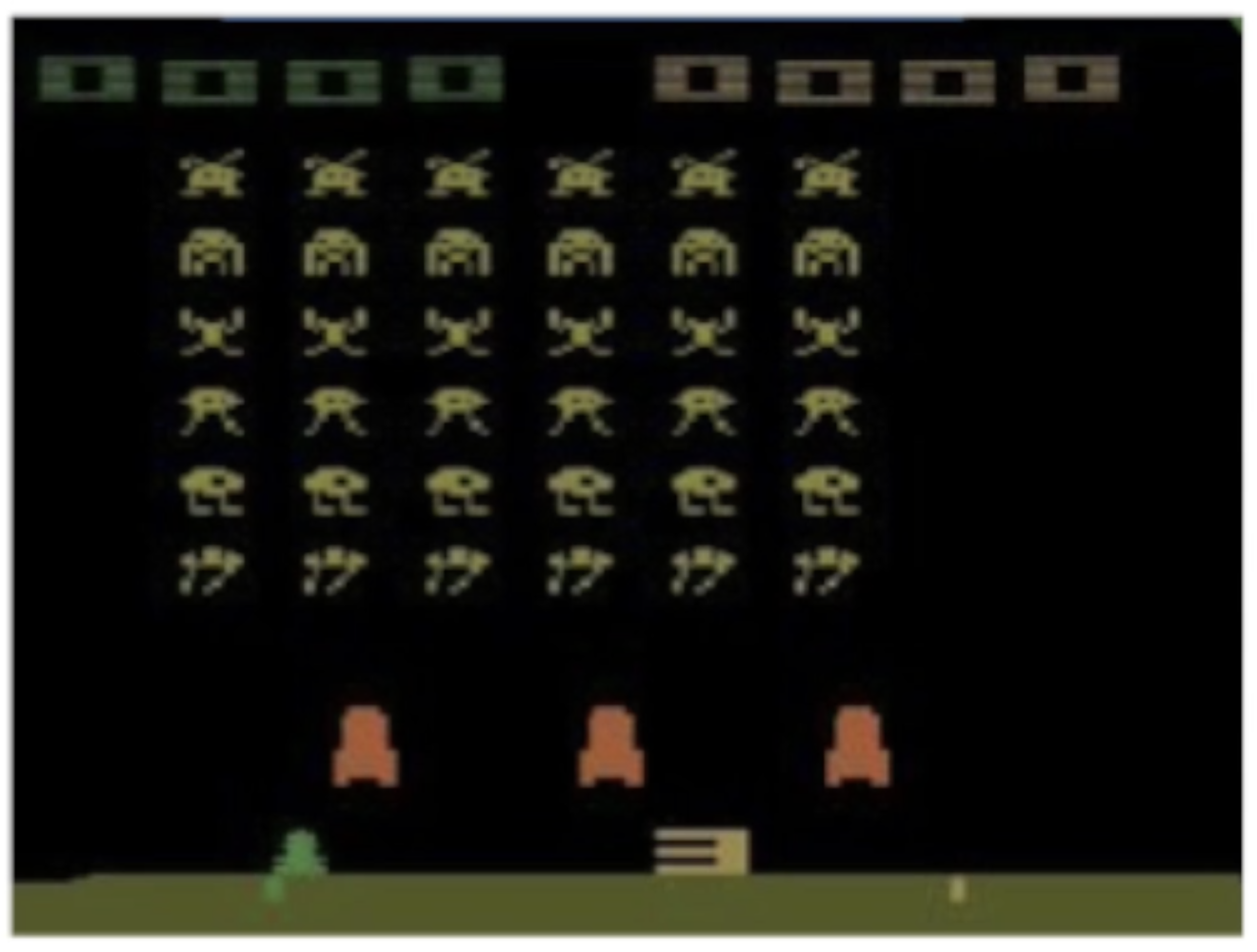to every other state:Let’s see the results! Notice how the metric jumps when an alien ship is destroyed: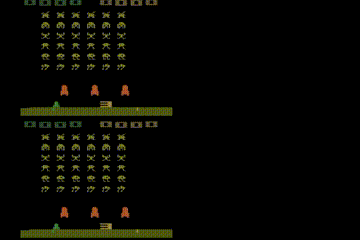## Citing

The original tweet is here.

Please use the following BibTeX entry if you’d like to cite this work:

@inproceedings{castro20bisimulation,
author    = {Pablo Samuel Castro},
title     = {Scalable methods for computing state similarity in deterministic {M}arkov {D}ecision {P}rocesses},
year      = {2020},
booktitle = {Proceedings of the Thirty-Fourth AAAI Conference on Artificial Intelligence (AAAI-20)},
}